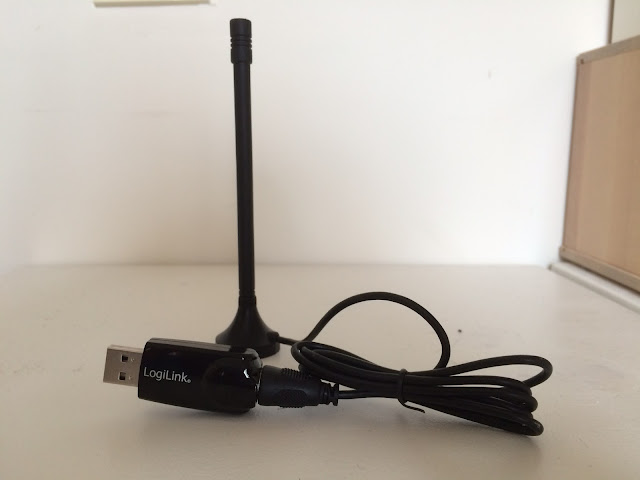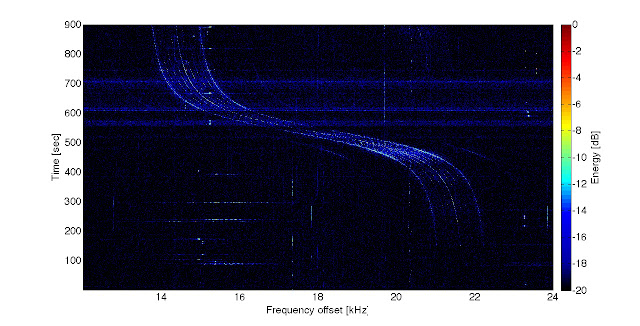## dinsdag 27 oktober 2015

### How to make a spectrogram or waterfall plot

In a few blogpost ago, I showed you several figures of a satellites transmission. You know, those beautiful S-curve plots with all the colours.  Those are called spectrograms or we like to call them waterfall plots, because the radio transmissions streams up in time like a reversed waterfall. To see such a waterfall plot live in action, the University of Twente opened their antennas for you to listen and see (see link). Yet, how to make such a figure?Logilink DVB-T USB stick with antenna works like a SDR and it is so small.
No back to the radio frequency spectrogram construction (OK, that also sounded nerdish), I have made a 6-step plan to convert the raw radio signal into a spectrogram.

1.     Check that the dataset is a complex signal;
2.     Define length of signal, sampling-rate, and center frequency;
3.     Define the time-step for the waterfall plot (trade-off between freq. & time resolution);
4.     Perform FFT for a segment of length equal to the times-step, iterate through complete signal;
5.     Represent the results in a waterfall plot;
6.     Choose color scale to better visualise signal.

Step 1: Check that the dataset is a complex signal
From the SDR-device or the USRP in the tracking station the data files are stored in the .fc32 format, which is a binary representation of a complex signal. You need the complex signal, because then the Fast Fourier Transform (FFT) will generate a full band representation of the signal. If the signal would be real (so not complex), only half of the spectrum would be visible. You would get two mirrored spectra. Here, a small MATLAB code to read the data into an matrix you can manipulate:

f = fopen ([filename '.32fc'], 'rb');
t = fread (f, [2, Inf], 'float');
v = t(1,:) - t(2,:)*1i;
[r, c] = size (v);
v = reshape (v, c, r);

You can set the count in fread to Inf, such that the complete file is read or a part of the file and iterate through the data. In the end you will have a complex vector, v, that contains the complete information of the recorded signal.

Step 2: Define length of signal, sampling-rate, and center frequency
For our recordings mostly we use 900 seconds of recording time, this is the average time for a satellite pass, the tuning frequency for Delfi-C3 is 145.870 MHz, and we use a sampling rate of 250 kHz, because of hardware specifications of our USRP. We also downsample the data to 50 kHz, which is still usable and takes up less memory space: 250 kHZ file is 1.7 Gb and 50 kHz file is 360 Mb.

Step 3: Define time-step for spectrogram
Here, you can play with time and frequency resolution. For now our aim is to have high accurate frequency determination every second, so we use Dt = 1 sec. You can play around with it, of course. Be careful with the memory of your computer, many times I have pushed my computer over the memory edge.

Step 4: Perform the FFT on the small defined part of the signal and iterate
The Fast Fourier Transform algorithm is a powerful piece of software. It can be very fast when handled properly. MATLAB has its inbuilt FFT algorithm, which works extremely fast. For Python there are many different options (SciPy version is unfortunately not as fast as MATLAB), and of course any well respected programming language should have the FFT algorithm implemented.

The FFT algorithm has a parameter that tells how to bin the calculated frequencies. In MATLAB this parameter is called NFFT. Always have this parameter higher than the length of your sample that is inputted in the FFT algorithm to get high frequency resolution. To increase performance of the FFT algorithm, adjust NFFT such that it is an exponential of 2. Then start iterate through the signal:

for i = 1:endSignal
% Select certain part of the signal
b = 1+ (i-1)*lengthPart;
e = lengthPart + (i-1)*lengthPart;
SigPart = v(b:e);
% Perform FFT algorithm on selected part
Y = fft(SigPart,NFFT);
line = 2*abs(Y(1:NFFT))./lengthPart
% Store the FFT result in Waterfall matrix
Waterfall(i,:) = line;
end

In the end you will get a matrix filled with frequency information from the recorded signal.

Step 5/6: Represent the Waterfall plot / choose appropriate color scale
The data is now in a matrix that you can plot with MATLAB using imagesc, or plot it to a .bmp file. Choose the option that works for your purpose and software. Lets see one of my results.Spectrogram of a Delfi-C3 pass for a 15 min recording at 145.870 MHz using a sampling-rate of 250 kHz.
By changing the colorbar, you can visualise the satellite signal much better. A beautiful S-curve due to the Doppler effect. Good luck!